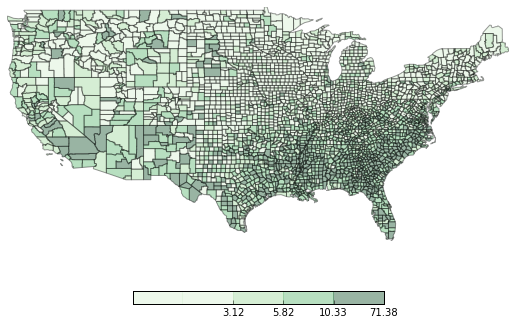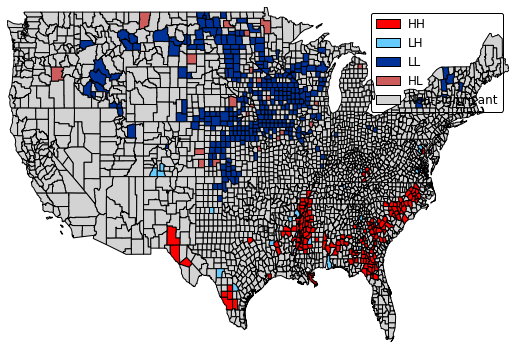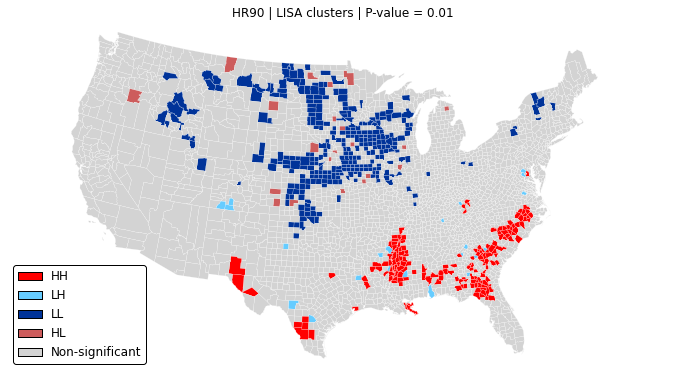# LISA cluster maps with PySAL¶

This notebook is available as a gist, or viewable online as static html.

Local Indicators of Spatial Association (LISAs, Anselin, 1995) are a common tool to explore spatial heterogeneity, identify spatial concentration of (dis)similar values and test for the probability such agglomerations originate from a random process.

PySAL has had functionality to run LISAs since its very beginning. However, the output of such computation is not very useful just by itself as, being a local statistic, LISAs imply running a test for every single observation. The usual approach has been to visualize significant clusters on a map using a simple color coding for each class (High-High, HH; Low-Low, LL; High-Low, HL; Low-High, LH). Such visualiations have been available for a long time in packages such as GeoDa, but this would imply breaking the workflow to switch between Python and GeoDa, plus with the annoyance that the workflow cannot thus be automated, a convenient feature for reproducible science.

In this notebook, we show how to leverage the visualization layer that is being built in PySAL to create generic and custom LISA cluster maps.

## Set up¶

For this example, we will use the NAT dataset, included in PySAL by default, exploring the variable HR90, homicide rates in 1990 at the county level.

Let's start by importing the required code. This is all code that will be made available in the upcoming release of PySAL in July 2015 (if you're reading this afterwards, it's all in there by default!).

In :
%matplotlib inline

import pysal as ps
import numpy as np
from pysal.contrib.viz import mapping as maps


Reading from  /Users/dani/code/pysal_darribas/pysal/examples/nat/NAT.shp


Before any further analysis, it is always good practice to visualize the distribution of values. We can now conveniently do that in PySAL:

In :
_ = maps.plot_choropleth(shp_link, hr90, 'quantiles', cmap='Greens', figsize=(9, 6))Before we can plot a map, we need to run a LISA. In the spirit of flexibility and modularity envisioned in PySAL, the two operations (computation, visualization) are decoupled. We will use a simple contiguity matrix for this.

In :
w = ps.queen_from_shapefile(shp_link)
lisa = ps.Moran_Local(hr90, w, permutations=9999)


## LISA maps in one line of code¶

The simplest way to obtain a quick visualization of LISA results is with the "one-liner" plot_lisa_cluster:

In :
_ = maps.plot_lisa_cluster(shp_link, lisa, figsize=(9, 6))## (Prettier) LISA maps using cartopy¶

The previous map is a very quick, painless way to obtain a visualization of LISA results. This is incredibly useful for interactive work where one wants to quickly iterate to get a sense of the data. However, once the researcher settles on a particular visualiation, there's some "eye-candy" that can be applied that improves notably the final result.

In this section, we use the fantastic library cartopy to produce a publication-ready LISA plot. This, in part, uses some of the internal machinery in pysal.contrib.viz, combined with plain matplotlib functionality.

NOTE: besides PySAL, you will need cartopy installed in your system to reproduce this example.

In :
import matplotlib.pyplot as plt
import cartopy.crs as ccrs

In :
orig_crs = ccrs.PlateCarree()
projection = ccrs.LambertConformal()
p_thres = 0.01

polys = maps.map_poly_shp(shp)
polys = maps.base_lisa_cluster(polys, lisa, p_thres=p_thres)
polys.set_edgecolor('1')
polys.set_linewidth(0.2)
polys.set_transform(orig_crs)

f = plt.figure(figsize=(12, 8))

ax = plt.axes(projection=projection)
extent = [shp.bbox, shp.bbox, shp.bbox, shp.bbox]
ax.set_extent(extent, crs=ccrs.PlateCarree())
ax.outline_patch.set_visible(False)

boxes, labels = maps.lisa_legend_components(lisa, p_thres=p_thres)
plt.legend(boxes, labels, loc='lower left', fancybox=True)

plt.title('HR90 | LISA clusters | P-value = %.2f'%p_thres)

plt.show()Having this kind of maps produced in an automated way is handy because it is very straightforward then to plot a series of them. As an example, we will plot a different map for solutions that consider different significance thresholds.

In :
orig_crs = ccrs.PlateCarree()
projection = ccrs.LambertConformal()
p_thresS = [0.1, 0.05, 0.01, 0.001]

f = plt.figure(figsize=(16, 10))

for i, p_thres in enumerate(p_thresS):
polys = maps.map_poly_shp(shp)
polys = maps.base_lisa_cluster(polys, lisa, p_thres=p_thres)
polys.set_edgecolor('1')
polys.set_linewidth(0.2)
polys.set_transform(orig_crs)

ax = plt.subplot(2, 2, i+1, projection=projection)
extent = [shp.bbox, shp.bbox, shp.bbox, shp.bbox]
ax.set_extent(extent, crs=ccrs.PlateCarree())